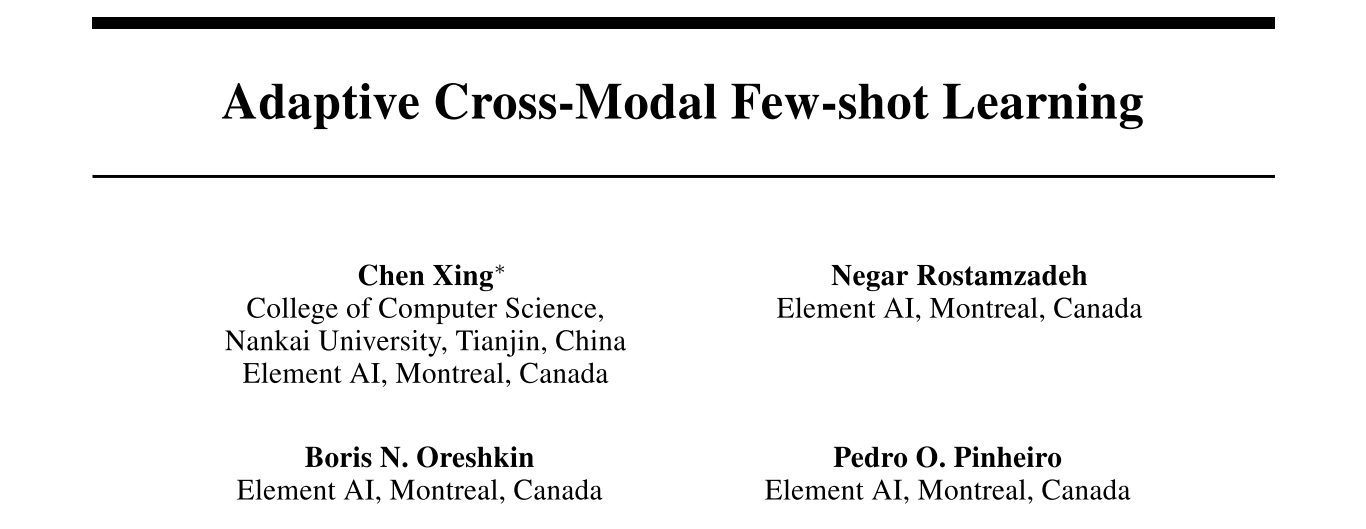## 论文信息

• 作者：Chen Xing, Negar Rostamzadeh, Boris N. Oreshkin, Pedro H. O. Pinheiro
• 出处：NeurIPS 2019
• 机构：Element AI, Montreal, Canada; Nankai University
• 关键词：few-shot learning, metric learning, multimodal
• 论文链接
• 开源代码：ElementAI/am3
• 其他资料：

## 内容简记

### 背景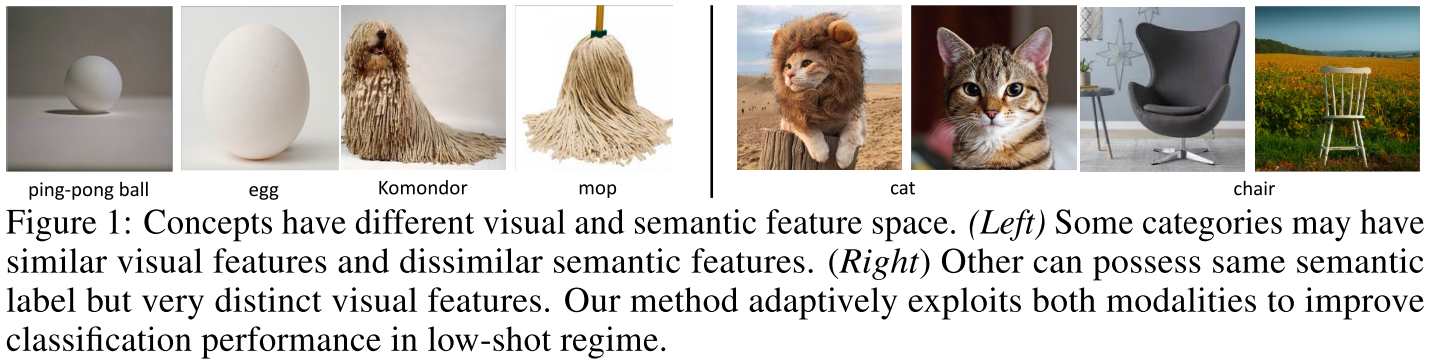### 方法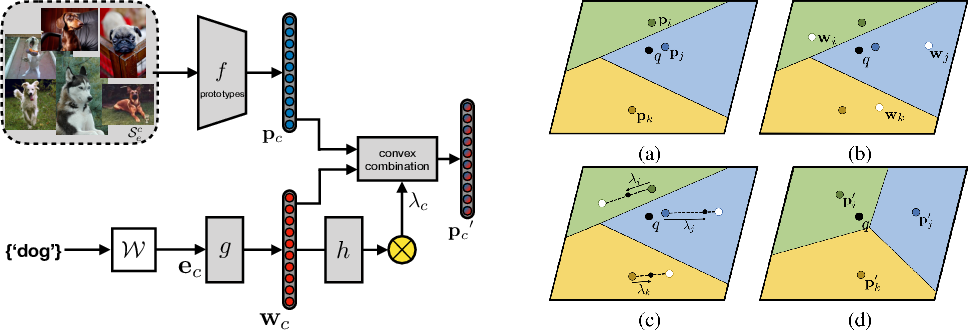$$\mathbf{p}_{c}^{\prime}=\lambda_{c} \cdot \mathbf{p}_{c}+\left(1-\lambda_{c}\right) \cdot \mathbf{w}_{c}$$

$$\lambda_{c}=\frac{1}{1+\exp \left(-h\left(\mathbf{w}_{c}\right)\right)}$$

$h$ 是自适应混合网络（adaptive mixing network）。自适应混合系数 $\lambda_{c}$ 可以根据不同的变量进行控制。

$$p_{\theta}\left(y=c | q_{t}, S_{e}, \mathcal{W}\right)=\frac{\exp \left(-d\left(f\left(q_{t}\right), \mathbf{p}_{c}^{\prime}\right)\right)}{\sum_{k} \exp \left(-d\left(f\left(q_{t}\right), \mathbf{p}_{k}^{\prime}\right)\right)}$$

$$\mathcal{L}(\theta)=\underset{\left(\mathcal{S}_{e}, \mathcal{Q}_{e}\right)}{\mathbb{E}}-\sum_{t=1}^{Q_{e}} \log p_{\theta}\left(y_{t} | q_{t}, \mathcal{S}_{e}\right)$$

### 实验

#### 对比实验

• miniImageNet 
• tieredImageNet 
• CUB-200 ：这是一个无样本学习数据集。考虑到大多数模态对齐方法没有在小样本学习数据集上有公开的实验结果，这个数据集用于将 AM3 与模态对齐方法进行对比

baselines：

• 单模态 FSL 方法：MAML、LEO 、原型网络、TADAM  等；
• 模态对齐方法：CADA-VAE  等；
• 模态对齐方法的基于度量的扩展：使用了基于度量的损失以及原型网络的训练方式（episode training）。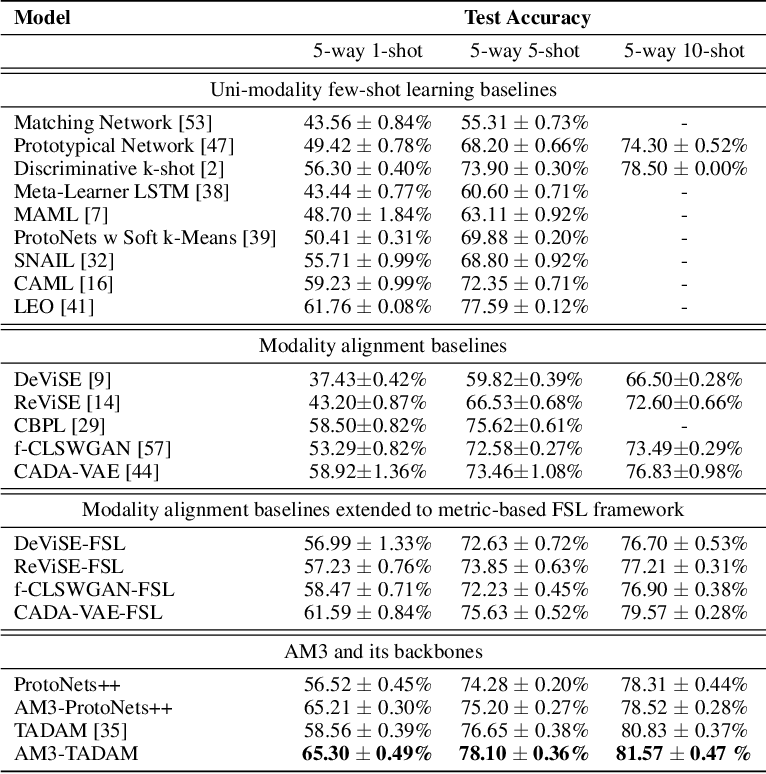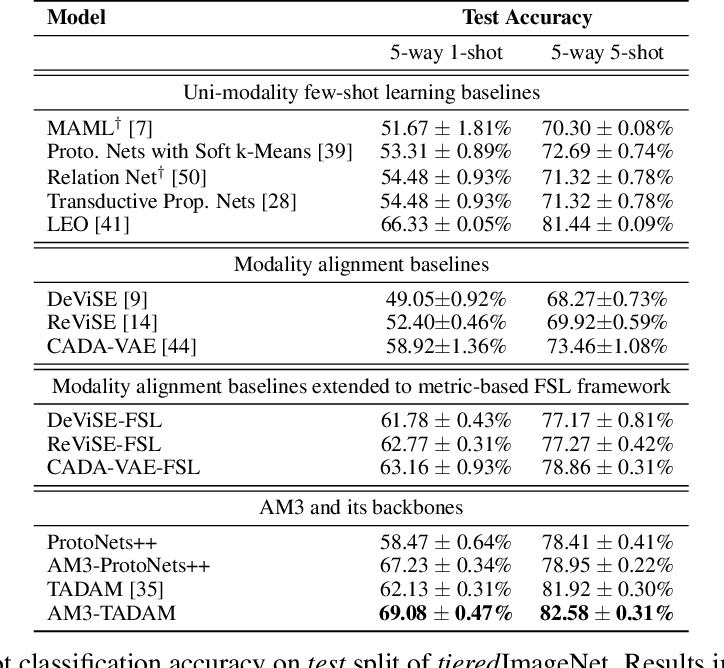1. 在所有数据集上，AM3 的表现都优于其主干方法。说明在正确使用时，文本模态可以帮助提高基于度量的 FSL 学习框架的性能；
2. AM3（主干方法选用 TADAM）取得 SOTA，且当 shot 数（即样本数）越少时，效果提升越多。说明当视觉模态提供的信息越少时，语义信息对分类的帮助越大；
3. 当扩展到基于度量、episodic、FSL 框架时时，所有模态对齐方法的表现都显著提升。然而扩展前后这些模态对齐方法都比单模态 FSL 方法的 SOTA 要差。说明尽管模态对齐方法对于 ZSL 中的跨模态是有效的，但并不适合 FSL。一个可能的原因是，两个不同的结构被迫对齐导致双方的一些信息可能损失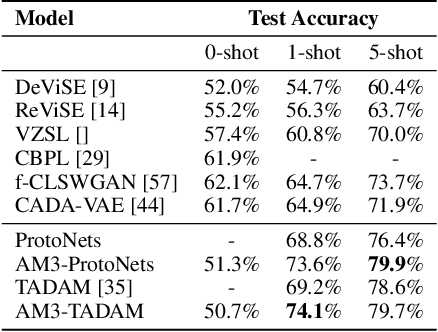#### 自适应机制分析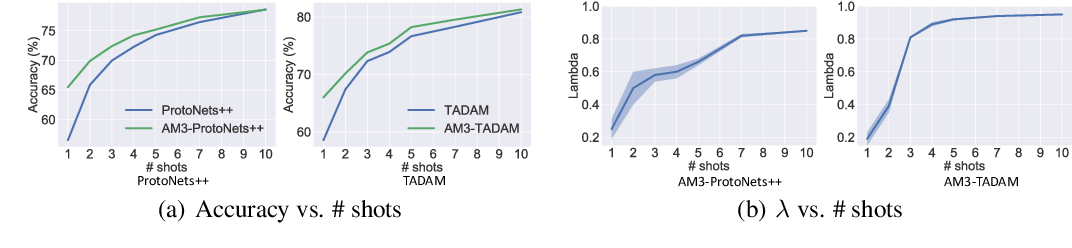1. 当 shot 数（即样本数）增加时，AM3 与其主干方法的表现差距减小；
2. 自适应混合系数 $\lambda_{c}$ 的平均值与 shot 数有关，当 shot 数减小时，AM3 在文本模态上的权重更大（在视觉模态上更小）。这种趋势表明，当视觉信息非常少时，AM3 可以自动将焦点更多地调整到文本模态以帮助分类；
3. 当 $\lambda_{c}$ 的方差随着 shot 数增加而减小时，AM3 与其主干方法的性能差距也会缩小。这表明 AM3 在类别级别的适应性对性能提升有非常重要的作用（？？？觉得有点牵强）。

#### 消融实验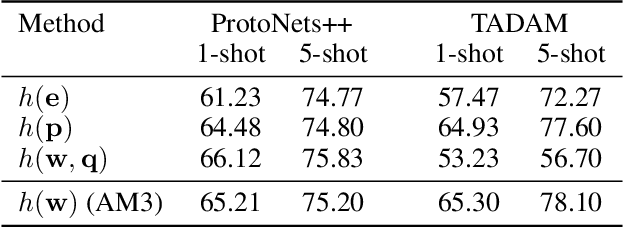## 参考论文

•  Zero-shot learning-the good, the bad and the ugly, CVPR 2017.
•  Matching networks for one shot learning, NIPS 2016.
•  Meta-learning for semi-supervised few-shot classification, ICLR 2018.
•  Caltech-UCSD Birds 200, Technical Report CNS-TR-2010-001, 2010.
•  Tadam: Task dependent adaptive metric for improved few-shot learning, NeurIPS 2018.
•  Meta-learning with latent embedding optimization, ICML 2016.
•  Generalized zero-and few-shot learning via aligned variational autoencoders, CVPR 2019.